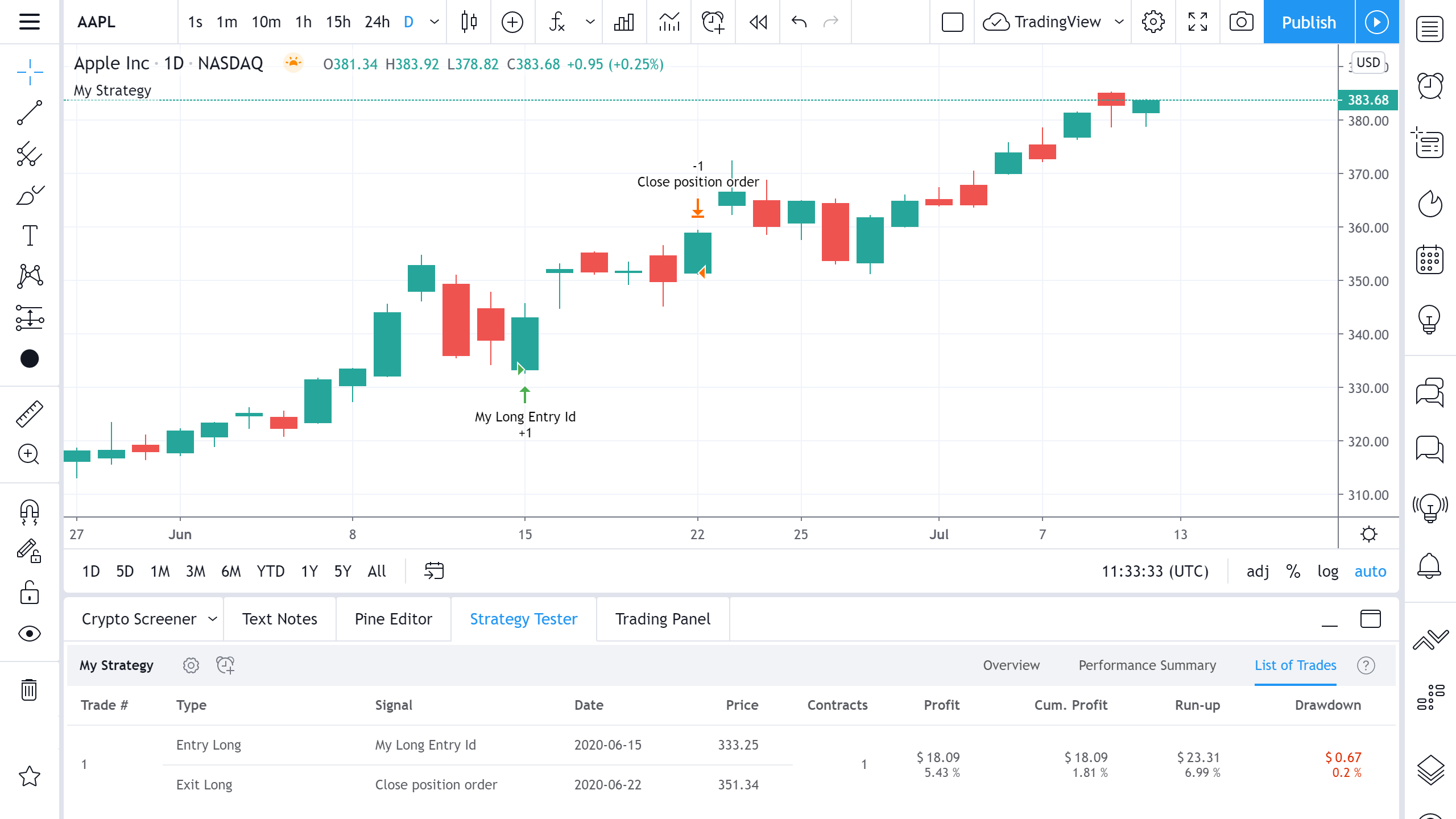# List Of Trades: Cumulative Profit

The cumulative profits or losses of the strategy after closing the trade. The percentage displays the relative gain/loss of the last trade as compared to the equity (initial capital + cumulative profit) before that trade.

Let's look at how the value for the Cumulative Profit column calculated:In this example, we bought 1 share of AAPL at the opening on June 15 and sold it at the opening of June 22. The initial capital was \$1000.

Cumulative profit is calculated by adding up all previous Cumulative Profit values with the current Profit value. In this case, we only have one trade, so the Cumulative Profit will be \$18.09, equal to Profit. Cumulative Profit Percentage value takes the Initial Capital into its calculation and is calculated with the formula: Cum. Profit % = Profit/(Initial Capital+Cumulative Profit of the previous trade)*100% = 18.09/(1000+0)*100 = 1.81%.